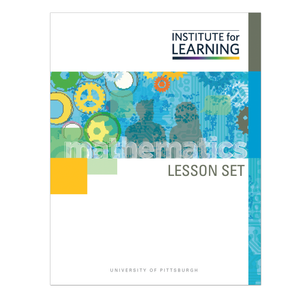# Investigating and Using Coordinate Geometry (Geometry)

Regular price \$6.25 \$0.00 Unit price per

In this lesson set, students explore coordinate geometry as a mechanism for proving simple geometric theorems and solving problems. They examine relationships between and among line segments and use the Pythagorean Theorem to develop common formulas associated with coordinate geometry. They learn how to generalize on the coordinate plane using (x1, y1) notation, and develop techniques for finding distance and dividing a segment into an a:b ratio.

Through engaging in this lesson set, students will:

• generalize midpoint and distance formulas using coordinate geometry;
• use coordinate geometry to explore patterns and make conjectures in the context of midpoint, distance, perpendicular bisectors, and midsegment; and
• use coordinate geometry to prove or disprove conjectures.

Standards: G-GPE.B.4, G-GPE.B.5, G-GPE.B.6, G-GPE.B.7